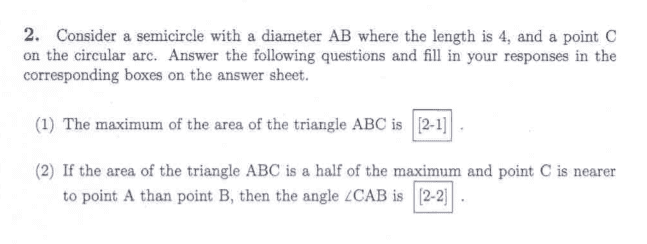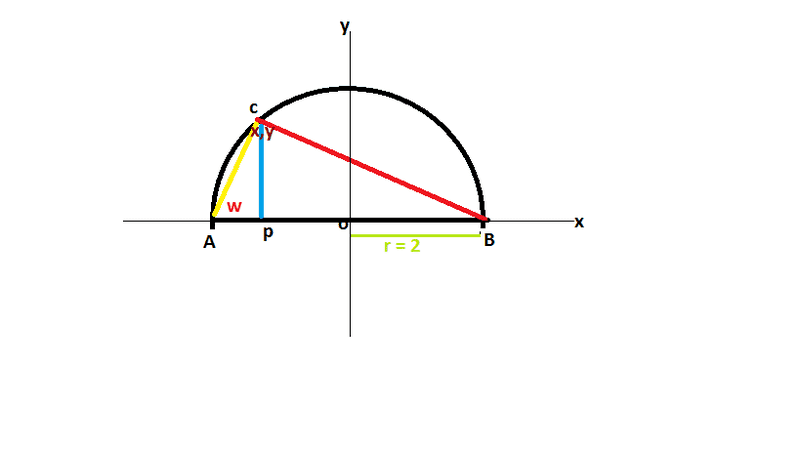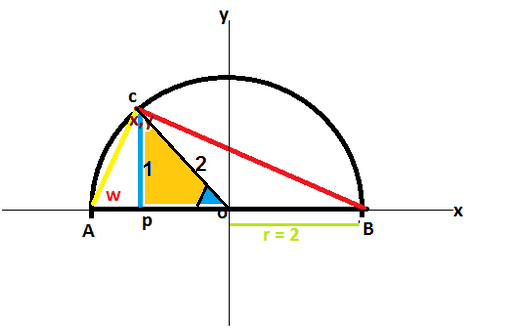# How to find the angles of a triangle in a semicircle?

• Helly123

## Homework Statement## Homework Equations

d(y)/d(x) --> max area
area of triangle = 1/2 . base . height

## The Attempt at a Solution

for number (2) [/B]x^2 + y^2 = r^2 --> circle equation
base = 2R, height = y
Area = 1/2 . 2R . y
area = 1/2 . 4. √ (r^2 - x^2)

area now is half of max = 2, so :
2 = 1/2 . 4. √ (r^2 - x^2)
2 = 2 √(4-x^2)
1 = √ (4-x^2)
1 = 4 - x^2
x = √3
now y = 1
AP = 2 - √3
I have to find w (angle of ACB)
PC = 1
how to find w ?

Consider the triangle AOC.

Consider the triangle AOC.
OC^2 = AC^2 + AO^2 -2AC.AO.cosw
4 = 4 + (8-4v3)^2 -8(8-4v3)cosw
Cos w = (8-4v3)/8
Cos w = 1-1/2v3

But the answer is in degrees, and not allowed using calculator.. And I don't think my answer is right.

Note: v3 = root 3

Consider the triangle AOC. You can find the angle at O by studying the right triangle CPO.

I don't get it.. Can anyone help??

I don't get it.. Can anyone help??CPO is a right triangle. You know the hypotenuse, it is one radius, length 2. You calculated PC, it is 1. What is the blue angle then?

•Helly123
View attachment 205500
CPO is a right triangle. You know the hypotenuse, it is one radius, length 2. You calculated PC, it is 1. What is the blue angle then?
The blue angle is 60degrees.

The blue angle is 60degrees.
No. Imagine half of an equilateral triangle, rotatedNo. Imagine half of an equilateral triangle, rotatedOh.. 30degrees ...

No. Imagine half of an equilateral triangle, rotatedSo, i get it. One triangle = 180degrees. I get 30, left 150. CAO = ACO. So CAO = 75 degrees?

No. Imagine half of an equilateral triangle, rotatedBut with theory, it should be sin w/ 2 = sin tetha / AC
I get sin w = 1/AC
While AC = 8-4root3
How can i get w with this method?

So, i get it. One triangle = 180degrees. I get 30, left 150. CAO = ACO. So CAO = 75 degrees?
Yes, it is correct.

But with theory, it should be sin w/ 2 = sin tetha / AC
What is theta, and what "theory" is that?
I get sin w = 1/AC
While AC = 8-4root3
How can i get w with this method?
Check with a calculator. Otherwise it is complicated.

•Helly123